# A slender 9 lb rod can rotate in a vertical plane about a pivot at B. A spring of constant k-30 lb/ft and of unstretched length 6 in. is attached to the rod as shown. The rod is released from rest in... related homework questions

• #### A slender 9 lb rod can rotate in a vertical plane about a pivot at B. A spring of constant k-30 lb/ft and of unstretched length 6 in. is attached to the rod as shown. The rod is released from rest in...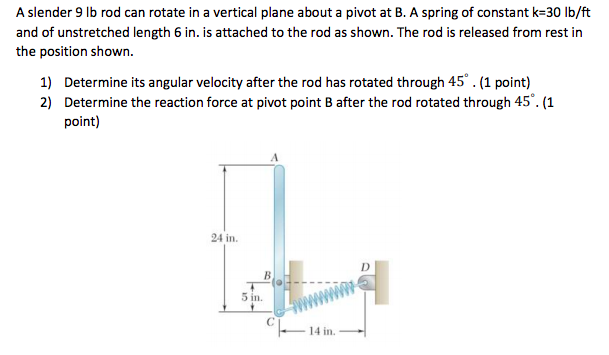A slender 9 lb rod can rotate in a vertical plane about a pivot at B. A spring of constant k-30 lb/ft and of unstretched length 6 in. is attached to the rod as shown. The rod is released from rest in the position shown. 1) Determine its angular velocity after the rod has rotated through 45.(1 point) 2) Determine...

• #### It’s review question, I need this as soon as possible. Thank you 3) For thè diferential equation: (a) The point zo =-1 is an ordinary point. Compute the recursion formula for the coefficients of...It’s review question, I need this as soon as possible. Thank you 3) For thè diferential equation: (a) The point zo =-1 is an ordinary point. Compute the recursion formula for the coefficients of the power series solution centered at zo- -1 and use it to compute the first three nonzero terms of the power series when -1)-s and v(-1)-0....

• #### Sample Problem 6. The 30 kg slender rod is released from rest when θ-00 (when rod OA is in the horizontal position). The spring is unstretched at θ-00. A constant clockwise moment of 10 N-m is applie...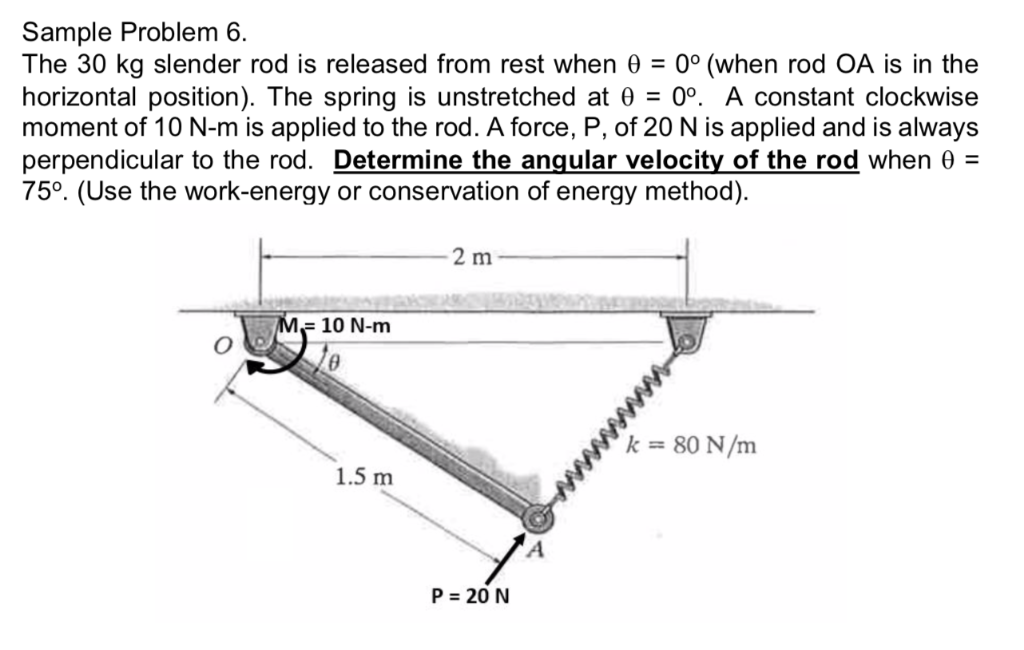Sample Problem 6. The 30 kg slender rod is released from rest when θ-00 (when rod OA is in the horizontal position). The spring is unstretched at θ-00. A constant clockwise moment of 10 N-m is applied to the rod. A force, P, of 20 N is applied and is always perpendicular to the rod. Determine the angular velocity of...

• #### Dont copié formé thé book oh ya dont copié formé thé book cause you Oiil inde up being triste soi remembré not toi copié frome thé book oh ya

Dont copié formé thé book oh ya dont copié formé thé book cause you Oiil inde up being triste soi remembré not toi copié frome thé book oh ya!translation in english please!

• #### A bead of mass m is welded at the periphery of the smoothly pivoted disc of mass m and radius R as shown in figure. The pivot axis is horizontal and through the centre of disc. The disc is free to rotate in vertical plane. The disc is released .......?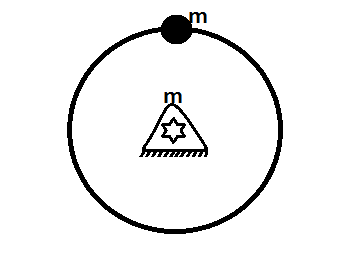A bead of mass #m# is welded at the periphery of the smoothly pivoted disc of mass m and radius #R# as shown in figure. The pivot axis is horizontal and through the centre of disc. The disc is free to rotate in vertical plane. The disc is released from rest from position shown. Find the speed of bead at...

• #### Homework Set # 9 Fundamental Problem 18.11 ▼ Part A The 60kg rod is released form rest when θ= 45°. (Figure 1) Determine the angular velocity of the rod when θ-0°. The spring is unstretched when 6-45...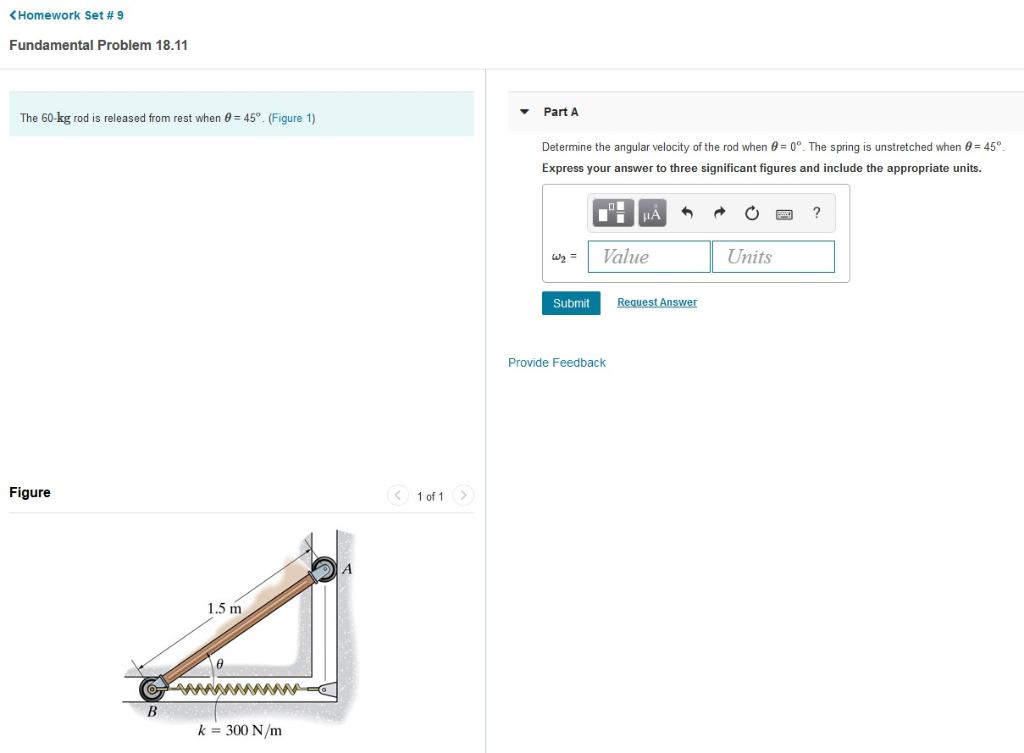Homework Set # 9 Fundamental Problem 18.11 ▼ Part A The 60kg rod is released form rest when θ= 45°. (Figure 1) Determine the angular velocity of the rod when θ-0°. The spring is unstretched when 6-45°. Express your answer to three significant figures and include the appropriate units. Value Units st Answer Submit Provide Feedback Figure 1.5 m k-300...

• #### A weightless rod of length 2L carries two equal mass 'm', one secured at lower end A and the other at the middle of the rod B. The rod can rotate in vertical plane?

What horizontal velocity must be imparted to the mass A so that it just completes the vertical circle?

• #### Problem 2: The uniform rod of length, L, and mass, m, shown below, is released from rest and from an angle of 8- O (radians]. The rod then begins to rotate clockwise due to the force of gravity m...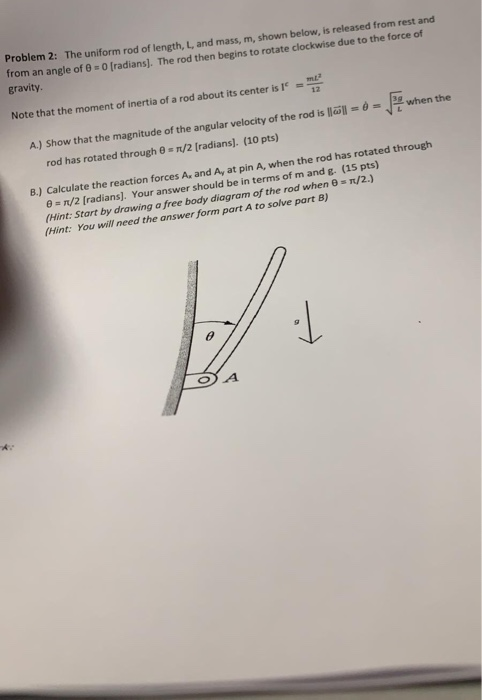Problem 2: The uniform rod of length, L, and mass, m, shown below, is released from rest and from an angle of 8- O (radians]. The rod then begins to rotate clockwise due to the force of gravity mE 12 Note that the moment of inertia of a rod about its center is when the A) Show that the magnitude...

• #### A 3.5-kg slender rod AB and a 2-kg slender rod BC are connected by a pin at Band by the cord AC. The assembly can rota...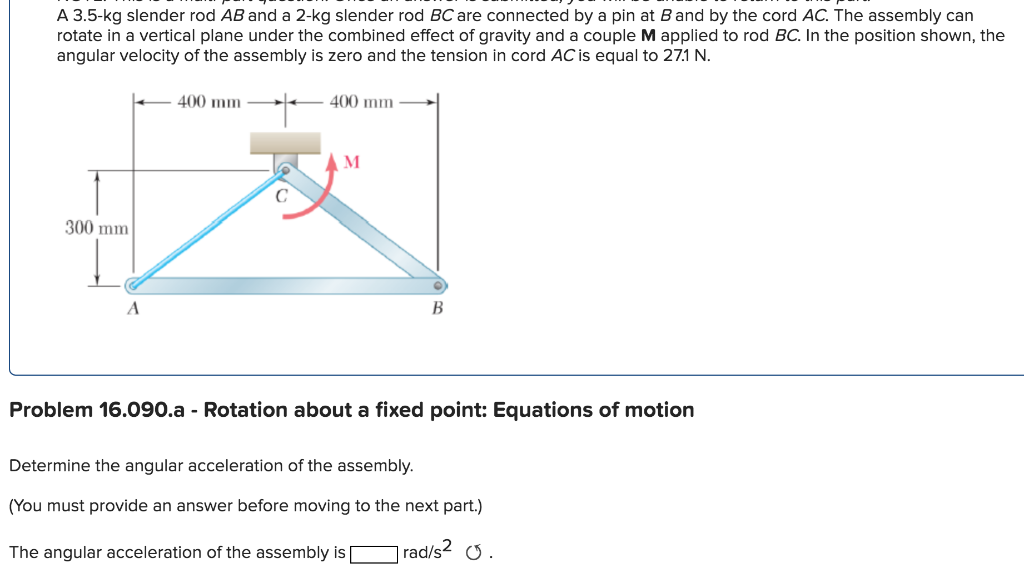A 3.5-kg slender rod AB and a 2-kg slender rod BC are connected by a pin at Band by the cord AC. The assembly can rotate in a vertical plane under the combined effect of gravity and a couple M applied to rod BC. In the position shown, the angular velocity of the assembly is zero and the tension in...

• #### A 12 kg slender rod is placed against the wall at an angle of 45 degrees as shown. If it is released from rest, determine the angular velocity of the rod at the instant just before it hits the gr...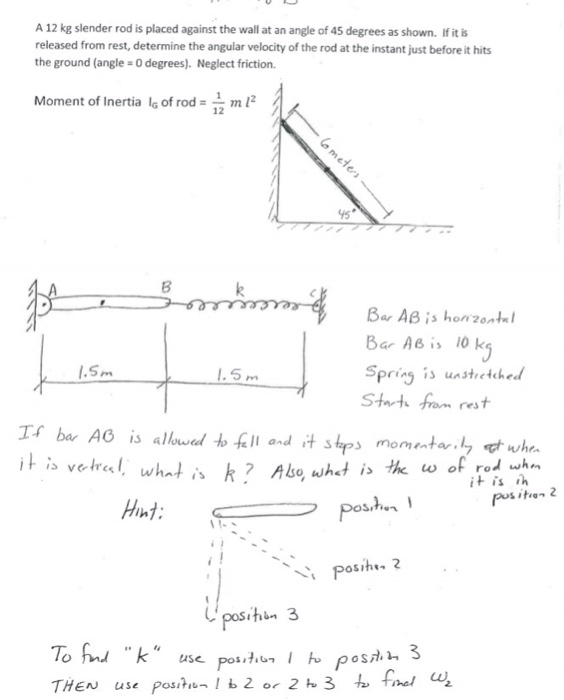A 12 kg slender rod is placed against the wall at an angle of 45 degrees as shown. If it is released from rest, determine the angular velocity of the rod at the instant just before it hits the ground (angle 0 degrees). Neglect friction. Moment of Inertia lg of rod-2 12 8 Bar AB is l0 k Spring unstrctched...

• #### A thin rod, of length L and negligible mass, that can pivot about one end to rotate in a vertical circle

A thin rod, of length L and negligible mass, that can pivot about one end to rotate in a vertical circle. A heavy ball of mass m is attached to the other end. The rod is pulled aside through an angle and released. (a)What is the speed of the ball at the lowest point if L = 2.20 m, =...

• #### An object of mass M = 4.00 kg is attached to a spring with spring constant k = 1100 N/m whose unstretched length is L...

An object of mass M = 4.00 kg is attached to a spring with spring constant k = 1100 N/m whose unstretched length is L = 0.170 m , and whose far end is fixed to a shaft that is rotating with an angular speed of ω = 5.00 radians/s . Neglect gravity and assume that the mass also rotates...

• #### An object of mass M = 4.00 kg is attached to a spring with spring constant k = 1100 N/m whose unstretched length is L...

An object of mass M = 4.00 kg is attached to a spring with spring constant k = 1100 N/m whose unstretched length is L = 0.170 m , and whose far end is fixed to a shaft that is rotating with an angular speed of ω = 5.00 radians/s . Neglect gravity and assume that the mass also rotates...

• #### An object of mass M = 2.00 kg is attached to a spring with spring constant k = 550 N/m whose unstretched length is L =...

An object of mass M = 2.00 kg is attached to a spring with spring constant k = 550 N/m whose unstretched length is L = 0.200 m , and whose far end is fixed to a shaft that is rotating with an angular speed of ω = 5.00 radians/s . Neglect gravity and assume that the mass also rotates...

• #### DSuppose \$39oo is deposited in a savings account that increases exponentially.Detamine thě APv if the acount...DSuppose \$39oo is deposited in a savings account that increases exponentially.Detamine thě APv if the acount increases to \$t020 in 4 years. Ass ume tne interest Vale remains Constant and no additional deposits or Withdrawals are made. (a.) Let pbe the APY. Note tnat if tme inital balaqe is yo, ne year later tne balane is %more. P- 3 (Tpe...

• #### A vertical spring (ignore its mass), whose spring constant is 875 N/m , is attached to a table and is compressed down by 0.160 m. What upward speed can it give to a 0.320-kg ball when released?

A vertical spring (ignore its mass), whose spring constant is 875 N/m , is attached to a table and is compressed down by 0.160 m. A) What upward speed can it give to a 0.320-kg ball when released? B) How high above its original position (spring compressed) will the ball fly?

• #### A vertical spring (ignore its mass), whose spring constant is 875 N/m , is attached to a table and is compressed down by 0.160 m. What upward speed can it give to a 0.320-kg ball when released?

A vertical spring (ignore its mass), whose spring constant is 875 N/m , is attached to a table and is compressed down by 0.160 m. A) What upward speed can it give to a 0.320-kg ball when released? I tried 7.88 m/s, but it said "Review your calculations; you may have made a rounding error or used the wrong number...

Need Online Homework Help?